A car moving in a circle is
always turning in the same direction

Penn State Schuylkill

Go Nittany Lions!!Ex 1) Circular motion horizontal surface w/ friction

A car with mass M moves in a circle of radius R on a asphalt road (µ) with a speed V.

Find V

Fc = mv2/r = µmg

v2/r = µg

v = (µgr)-1

Ex 2) Mass on a String - Vertical Circle

Mass M attached to a string moves at constant speed of v in a vertical circle of radius r.

Find string tension Ttop and Tbottom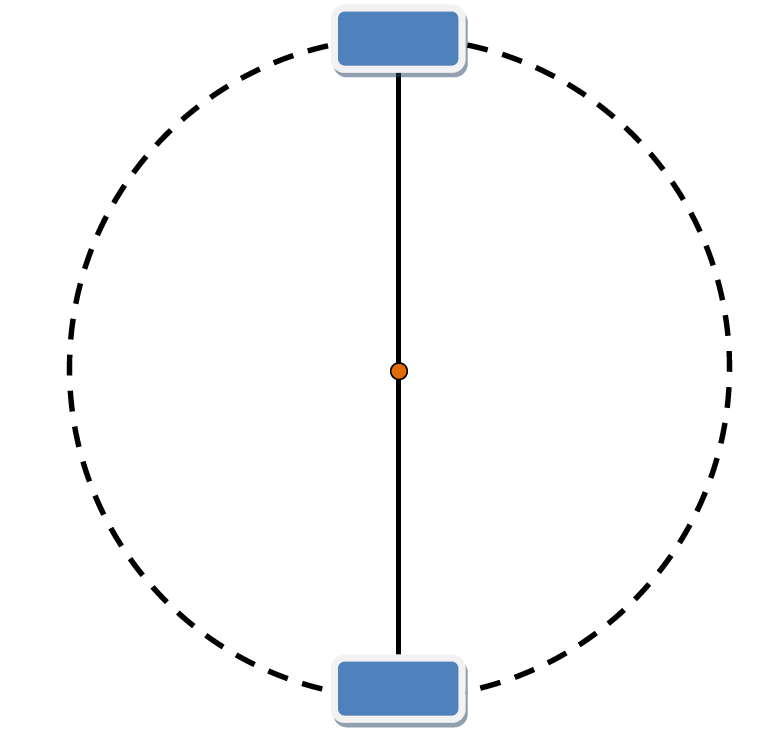Top

Free Body Diagram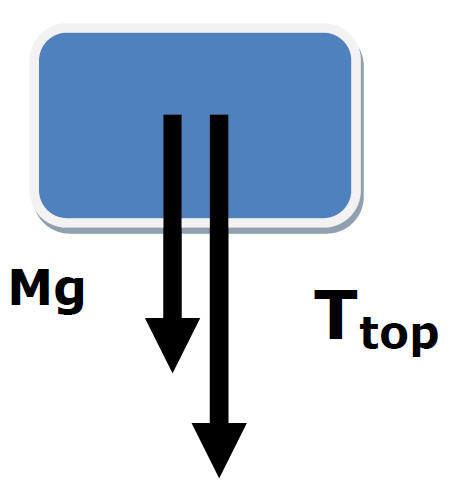Make center of circle +

Fnet = ma

Fnet = Mg + Ttop

Mv2/r = Mg + Ttop

M[v2/r - g] =  Ttop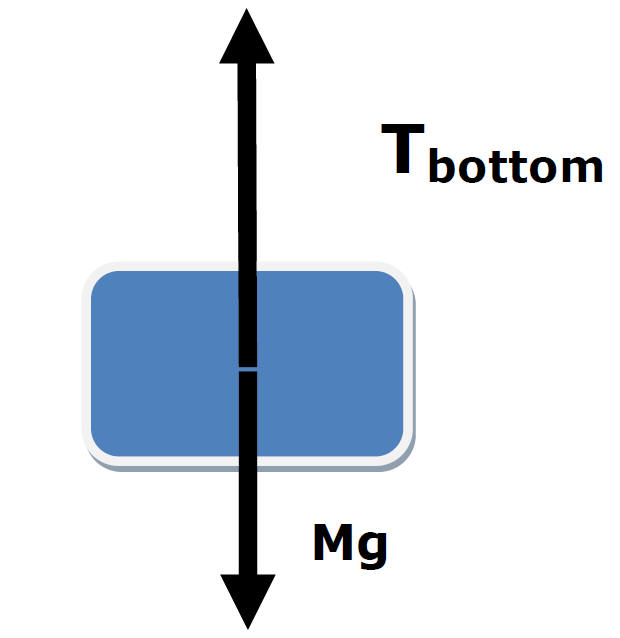Fnet = ma

Fnet = Tbottom - Mg

Mv2/r = Tbottom - Mg

M[v2/r + g] = TbottomMv2/r = Mg + Ttop

Mv2/r = Tbottom - Mg

Since v is constant ...

Mg + Ttop = Tbottom - Mg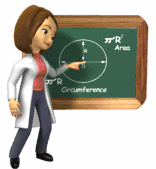2Mg + Ttop = Tbottom

Hammer Throw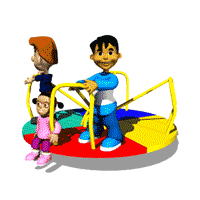Ex 3) Object mass M moves at constant speed V at the end of string in a circle r.

What is the minimum speed needed to maintain the object's circular motion?

What is Ttop at minimum speed?

Ttop = 0

Free Body DiagramMake center of circle +

Fnet = ma

Fnet = Mg + Ttop

Mv2/r = Mg

When Ttop = 0

v2/r = g

Ex 4) Rollercoaster - velocity changes with height

Car of mass M, circle radius r,
speed v

Find Normal Force at top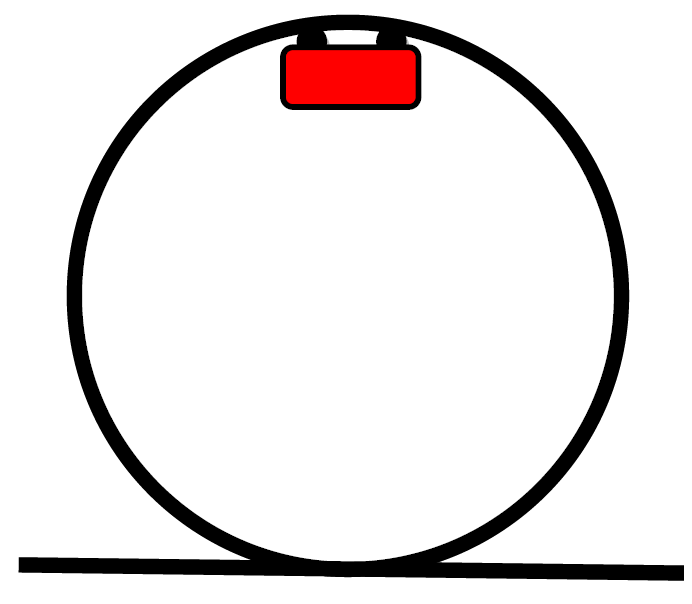Freebody diagram at top:

Fnet = mv2/r

Fn + mg = mv2/r

Fn = [mv2/r] - mg

What minimum velocity is required for the roller coaster to prevent the passengers from falling off?Minimum v occurs when Fn = 0

Fn = [mv2/r] - mg

[mv2/r] = mg

v2/r = gc Banked Track